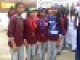# What is a force?

Show/Hide Sub-topics (Forces & Turning Effect Of Forces | O Level)
Show/Hide Sub-topics (Forces And Dynamics | A Level)

A force can be a push or a pull. A force acting on a body tend to produce a change in velocity or acceleration of the body.

• SI unit of force is the newton (N). It is a vector quantity.
• Whenever we are pushing, pulling, lifting, bending, twisting, tearing, stretching or squeezing, we are exerting a force.

## What can a force do?

A force can:

• make a stationary body move
• change the speed of a body
• change the direction of motion of a body (It is possible to change the direction of motion of a body without changing its speed.)
• change the size or shape of the body

## Main Types of forces

### Gravitational Force

• An attractive force (gravitational pull) that is only dependent on the masses of the objects.
• Long range force which acts at a distance
• Can usually be neglected unless one of these masses is very large

### Electromagnetic Forces

• Exist between moving or stationary electrical charges
• Can be an attractive or repulsive force

### Nuclear Forces/ Strong Force

• Forces between protons and neutrons and other sub-nuclear particles when they are close together
• Holds the atomic nucleus together as it keeps the protons together in the nucleus, despite their mutual electromagnetic repulsion

### Weak Force

• Responsible for radioactive decay and neutrino interactions
• Short range and very weak

## Other Types of Forces

### Contact Force

Contact force is a force perpendicular to the surface experienced by a body when it is in physical contact with something else.

This happens when atoms of one object get too close to the atoms of another object. The contact forces are actually manifestations(signs) of the electromagnetic force.

• Example: When you press your hand against your desk, you feel the repulsion of the electrons in the atoms of your hand against the electrons in the atoms of the desk.

### Frictional Force

• Acts in the opposite direction to the direction of motion of the body
• Resistive force acting between bodies that tends to oppose motion

#### For moving objects,

• Contact force now consists of Normal contact force (N) and Frictional force (f)
• Contact force is the resultant of N and f

### Viscous Force (Drag Force)

• When a solid moves in a fluid, it will experience a resistance to its motion
• Dependent on the speed of the object
• For objects moving at low speeds, viscous force is proportional to its speed
• For objects at high speeds, the viscous force is proportional to the square of the speed of the car
• Depends on the fluid involved
• Higher viscosity in denser fluids
• Dependant on nature of fluid

### Tension force

• Bi-directional force
• Always directed away from the objects and along the length of the string

## More on Contact Force

As shown in the picture above, an object resting on a table will experience a contact force (or known as normal force) acting on it in the upward direction.

Notice that the box is at rest. From Newton’s First Law of Motion (you will learn about this law in the subsequent sub-topics), you will know that this means that the net resultant force on the object is zero (i.e. all the forces are balanced). Hence, the normal force and the weight are of equal magnitude (that are acting in the opposite direction).

The normal force starts at the base of the box, where there is contact between the box and the table.

Weight starts from the center of mass of the box (indicated by $C.M.$).### 4 thoughts on “What is a force?”

1.In Last example how would we justify Newtons Third Law. Because, the point of force for both forces (Normal and weight force) are changed.

2.this was really helpful but u should include more details

•3.